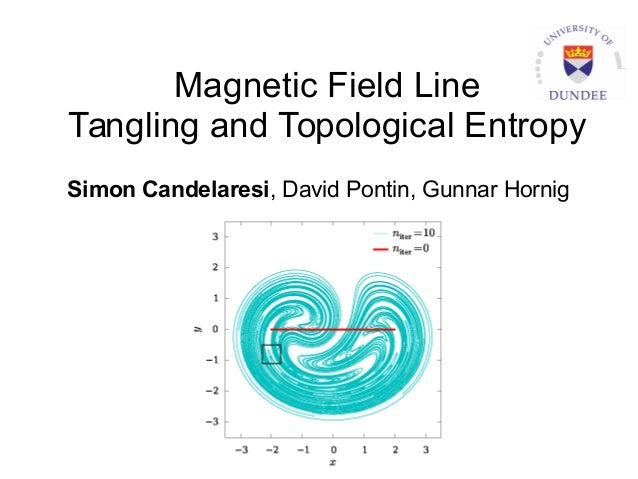Successfully reported this slideshow.Upcoming SlideShare
×

# Magnetic Field Line Tangling and Topological Entropy

395 views

Published on

Mixing of two-dimensional flows or three-dimensional magnetic fields is quantified using the finite time topological entropy FTTE. Similar to the finite time Lyapunov exponent it quantifies the amount of mixing of the fluid or chaotic dynamics in the system. Here we present an efficient method on how to compute the FTTE for periodic magnetic fields, like in Tokamaks, or for time periodic two-dimensional flows. Our method is both precise and highly time efficient. To show case the method we apply it to such cases that describe tangled or twisted magnetic fields.

Published in: Science
• Full Name
Comment goes here.

Are you sure you want to Yes No• Be the first to comment

• Be the first to like this

### Magnetic Field Line Tangling and Topological Entropy

1. 1. Magnetic Field Line Tangling and Topological Entropy Simon Candelaresi, David Pontin, Gunnar Hornig
2. 2. Magnetic Fields 2 NASA (Thiffeault et al. 2006) Field line tangling in solar and laboratory magnetic fields. Study the tangling of magnetic field lines.
3. 3. Topological Entropy Finite Time Topological Entropy (FTTE): Adaptive refinement: Number of necessary points large! 3
4. 4. Blinking Vortex Experiments Repeated applications of the blinking vortex motion. World lines correspond to 3d braided magnetic field. 4 twist parameter
5. 5. Blinking Vortex Experiments Adaptive refinement successfully increases resolution where needed. Greatly decrease number of points. 5 (Candelaresi et al. 2017)
6. 6. Blinking Vortex Experiments Speed up of 450x compared to previous methods. Accurately compute the FTTE 6 (Candelaresi et al. 2017)
7. 7. Topological Entropy Distribution 7 Map circles and measure their exponential stretching. FTTE distribution shows areas of chaotic behavior.
8. 8. Conclusions ● Measure field line tangling through topological entropy. ● Estimate the entropy through material line stretching. ● Adaptively refine calculations. ● Speed up of 450x. ● Spatial topological entropy distribution. simon.candelaresi@gmail.com